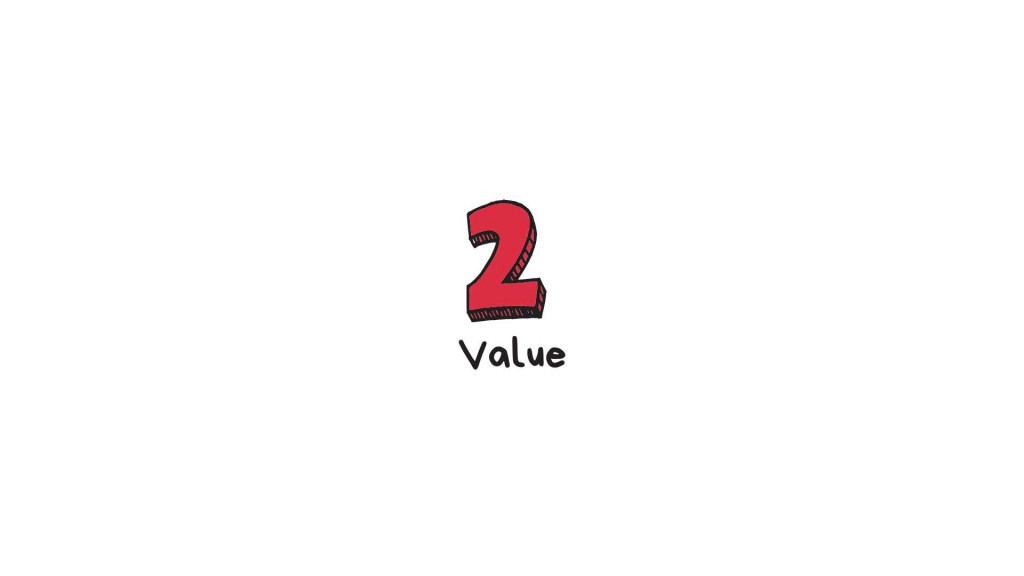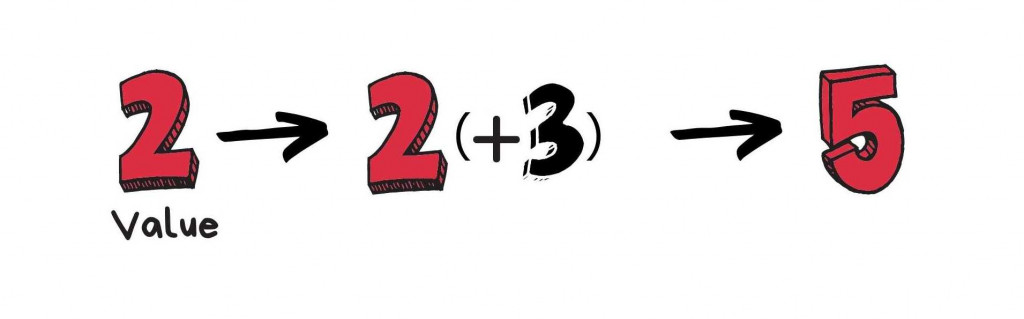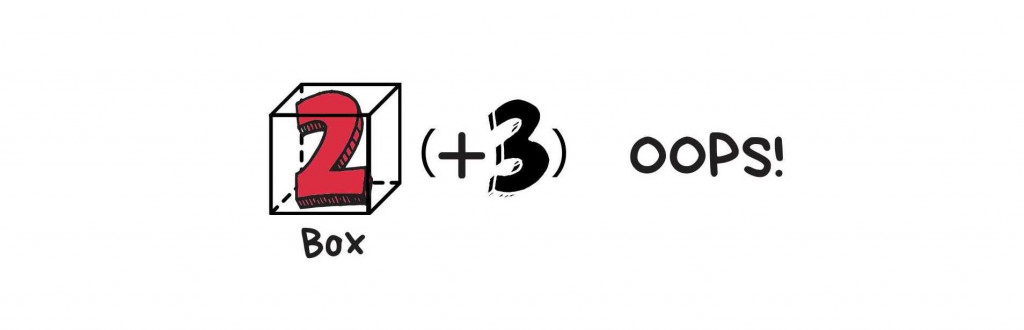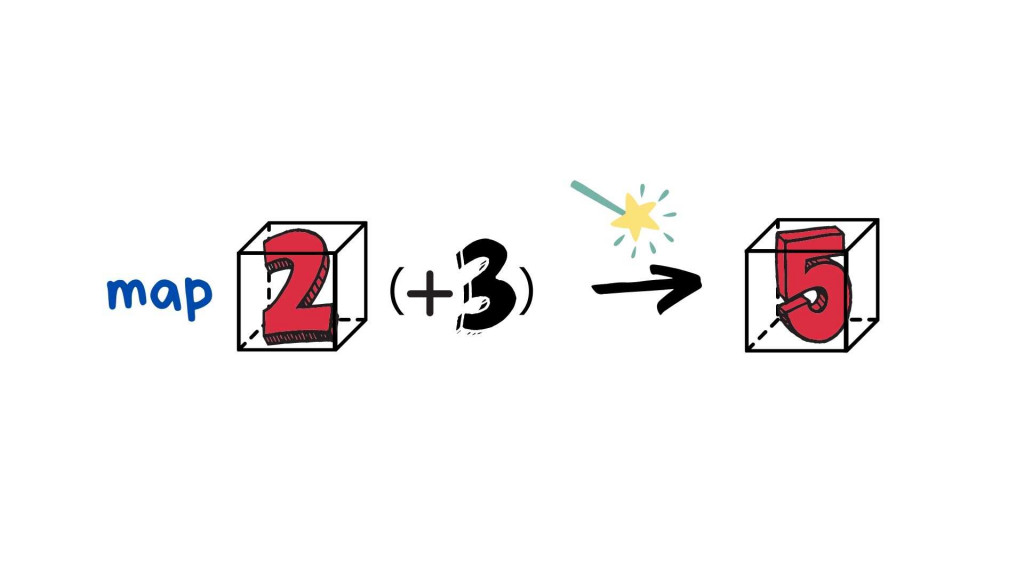#DAY 21
1
Software Development

## Functor 2: 圖解 Box Data Type### "The combination of calling map and the box tab type would lead we call functor"

Note. 接下來一系列的圖都是參考經典圖 Aditya Bhargava 然後為了加深印象再自己用 Canva 畫的。

# What's Box?``````const add = x => x + 3;
````````````const Box = x => ({
map: f => Box(f(x)), // 神奇的運算在此
fold: f => f(x), // 取值 remove from the box
});

const result = Box(2)
.map(x => x + 3) // Box(5)
```````map` 內部，實際上是這樣運作的: 取出值，用 function 處理過後再裝回 Context 裡

``````const Box = x => ({
map: f => Box(f(x)),
fold: f => f(x), // 取值 remove from the box
})
const result = Box(2)
.fold(x => x + 3) // 5
``````

# Why use Functor?

JS 本質仍不是一個 FP 語言，所以應用到了 Functor 的概念我只能盡量解釋，主要目的就是他可以讓 Value 維持在 Box 裡面，但仍然可以擁有一些 State 跟 method (像 OOP 的 class)。這樣讓 Box 自己運用函式也可以達成抽象化，我們並不是直接呼叫函式，而是說

Hey Box, call that function to me

``````/**
@parm {value} x
*/
const Box = x => ({
map: f => Box(f(x)),
value: x // 只是容易看值用而已
})

Box('a')
.map(x => x.toUpperCase())
.value;  //'A'
``````

`x => x.toUpperCase()``x` 在這邊用完之後就會完全消失，你不用煩惱現在 x 到底是多少，但 Class 的狀態會是一直存在的，需要去記憶他現在到底是多少。

``````// input 為 Array 寫法
['a']
.map(x => x.toUpperCase())

// ['A']
/**
@parm {value} x
*/
const Box = x => ({
map: f => Box(f(x)),
value: x // 只是容易看值用而已
})

Box('a')
.map(x => x.toUpperCase())
.value;  //'A'
``````

`map` 看起來像 recursive，但其實不是，他只是把運算過的值放回 Box 而已。

#### 參考文章

``````如有錯誤或需要改進的地方，拜託跟我說。

``````